# Water Distribution System Scheduling Using Reinforcement Learning

This example shows how to learn an optimal pump scheduling policy for a water distribution system using reinforcement learning (RL).

### Water Distribution System

The following figure shows a water distribution system.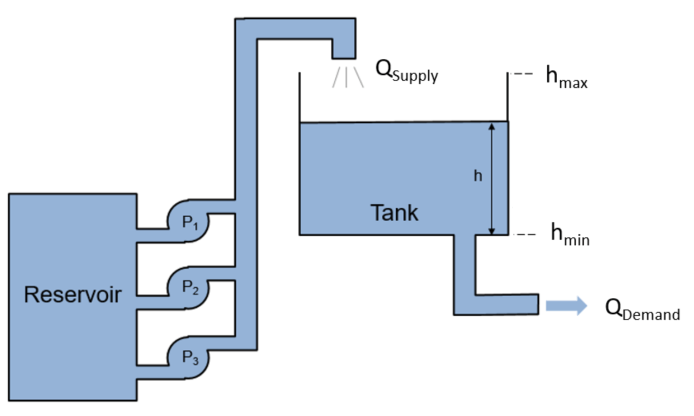Here:

• ${\mathit{Q}}_{\mathrm{Supply}}$ is the amount of water supplied to the water tank from a reservoir.

• ${\mathit{Q}}_{\mathrm{Demand}}$ is the amount of water flowing out of the tank to satisfy usage demand.

The objective of the reinforcement learning agent is to schedule the number of pumps running to both minimize the energy usage of the system and satisfy the usage demand ($\mathit{h}>0$). The dybnamics of the tank system are governed by the following equation.

`$\mathit{A}\frac{\mathit{dh}}{\mathit{dt}}={\mathit{Q}}_{\mathrm{Supply}}\left(\mathit{t}\right)-{\mathit{Q}}_{\mathrm{Demand}}\left(\mathit{t}\right)$`

Here, $\mathit{A}=40\text{\hspace{0.17em}}{\mathit{m}}^{2}$ and ${\mathit{h}}_{\mathrm{max}}=7\mathit{m}$. The demand over a 24 hour period is a function of time given as

`${\mathit{Q}}_{\mathrm{Demand}}\left(\mathit{t}\right)=\mu \left(\mathit{t}\right)+\eta \left(\mathit{t}\right)$`

where $\mu \left(\mathit{t}\right)$is the expected demand and $\eta \left(\mathit{t}\right)$ represents the demand uncertainty, which is sampled from a uniform random distribution.

The supply is determined by the number of pumps running, $\mathit{a}\in \left\{0,1,2,3\right\}$according to following mapping.

`${\mathit{Q}}_{\mathrm{Supply}}\left(\mathit{t}\right)=\mathit{Q}\left(\mathit{a}\right)=\left\{\begin{array}{ll}0& \mathit{a}=0\\ 164& \mathit{a}=1\\ 279& \mathit{a}=2\\ 344& \mathit{a}=3\end{array}\text{\hspace{0.17em}\hspace{0.17em}}\frac{\mathrm{cm}}{\mathit{h}}$`

To simplify the problem, power consumption is defined as the number of pumps running, $\mathit{a}$.

The following function is the reward for this environment. To avoid overflowing or emptying the tank, an additional cost is added if the water height is close to the maximum or minimum water levels, ${\mathit{h}}_{\mathrm{max}}$or ${\mathit{h}}_{\mathrm{min}}$, respectively.

`$\mathit{r}\left(\mathit{h},\mathit{a}\right)=-10\left(\mathit{h}\ge \left({\mathit{h}}_{\mathrm{max}}-0.1\right)\right)-10\left(\mathit{h}\le 0.1\right)-\mathit{a}$`

### Generate Demand Profile

To generate and a water demand profile based on the number of days considered, use the `generateWaterDemand` function defined at the end of this example.

```num_days = 4; % Number of days [WaterDemand,T_max] = generateWaterDemand(num_days);```

View the demand profile.

`plot(WaterDemand)`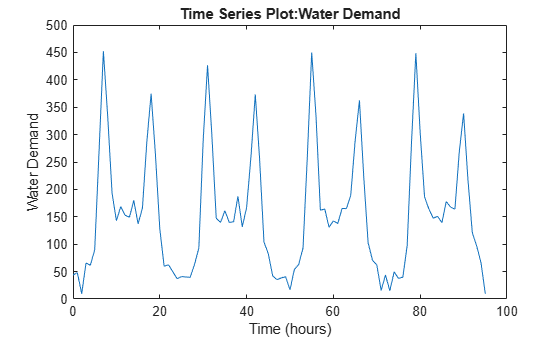### Open and Configure Model

Open the distribution system Simulink model.

```mdl = "watertankscheduling"; open_system(mdl)```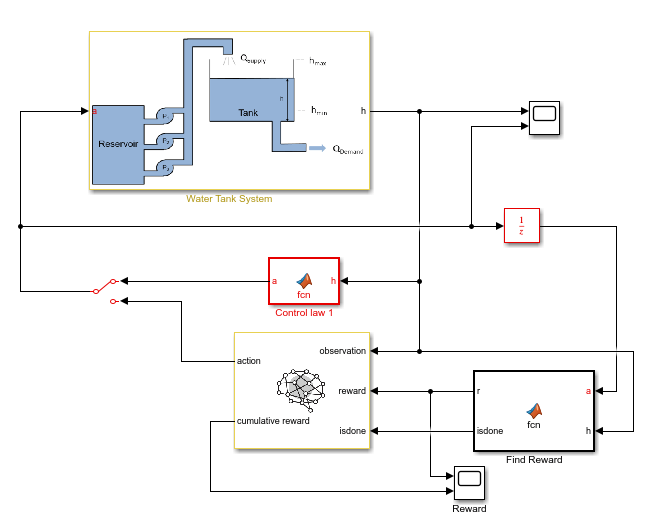In addition to the reinforcement learning agent, a simple baseline controller is defined in the Control law MATLAB Function block. This controller activates a certain number of pumps depending on the water level.

Specify the initial water height.

`h0 = 3; % m`

Specify the model parameters.

```SampleTime = 0.2; H_max = 7; % Max tank height (m) A_tank = 40; % Area of tank (m^2)```

### Create Environment Interface for RL Agent

To create an environment interface for the Simulink model, first define the action and observation specifications, `actInfo` and `obsInfo`, respectively. The agent action is the selected number of pumps. The agent observation is the water height, which is emasured as a continuous-time signal.

```actInfo = rlFiniteSetSpec([0,1,2,3]); obsInfo = rlNumericSpec([1,1]);```

Create the environment interface.

`env = rlSimulinkEnv(mdl,mdl+"/RL Agent",obsInfo,actInfo);`

Specify a custom reset function, which is defined at the end of this example, that randomizes the initial water height and the water demand. Doing so allows the agent to be trained on different initial water levels and water demand functions for each episode.

`env.ResetFcn = @(in)localResetFcn(in);`

### Create DQN Agent

A DQN agent approximates the long-term reward, given observations and actions, using a critic Q-value function representation. To create the critic, first create a deep neural network. For more information on creating a deep neural network value function representation, see Create Policy and Value Function Representations.

```% Fix the random generator seed for reproducibility. rng(0);```

Create a deep neural network for the critic. For this example, use a non-recurrent neural network. To use a recurrent neural netwrok, set `useLSTM` to `true`.

```useLSTM = false; if useLSTM layers = [ sequenceInputLayer(obsInfo.Dimension(1),"Name","state","Normalization","none") fullyConnectedLayer(32,"Name","fc_1") reluLayer("Name","relu_body1") lstmLayer(32,"Name","lstm") fullyConnectedLayer(32,"Name","fc_3") reluLayer("Name","relu_body3") fullyConnectedLayer(numel(actInfo.Elements),"Name","output")]; else layers = [ featureInputLayer(obsInfo.Dimension(1),"Name","state","Normalization","none") fullyConnectedLayer(32,"Name","fc_1") reluLayer("Name","relu_body1") fullyConnectedLayer(32,"Name","fc_2") reluLayer("Name","relu_body2") fullyConnectedLayer(32,"Name","fc_3") reluLayer("Name","relu_body3") fullyConnectedLayer(numel(actInfo.Elements),"Name","output")]; end```

Specify options for creating a critic representation.

`criticOpts = rlRepresentationOptions('LearnRate',0.001,'GradientThreshold',1);`

Create the critic representation using the defined deep neural network and options.

```critic = rlQValueRepresentation(layerGraph(layers),obsInfo,actInfo,... 'Observation',{'state'},criticOpts);```

### Create DQN Agent

To create an agent, first specify agent options. If you are using an LSTM network, set the sequence length to `20`.

```opt = rlDQNAgentOptions('SampleTime',SampleTime); if useLSTM opt.SequenceLength = 20; else opt.SequenceLength = 1; end opt.DiscountFactor = 0.995; opt.ExperienceBufferLength = 1e6; opt.EpsilonGreedyExploration.EpsilonDecay = 1e-5; opt.EpsilonGreedyExploration.EpsilonMin = .02;```

Create the agent using the defined options and critic representation.

`agent = rlDQNAgent(critic,opt); `

### Train Agent

To train the agent, first specify the training options. For this example, use the following options.

• Run training for 1000 episodes, with each episode lasting at `ceil(T_max/Ts)` time steps.

• Display the training progress in the Episode Manager dialog box (set the `Plots` option)

Specify the options for training using an `rlTrainingOptions` object.

```trainOpts = rlTrainingOptions(... 'MaxEpisodes',1000, ... 'MaxStepsPerEpisode',ceil(T_max/SampleTime), ... 'Verbose',false, ... 'Plots','training-progress',... 'StopTrainingCriteria','EpisodeCount',... 'StopTrainingValue',1000,... 'ScoreAveragingWindowLength',100);```

While you don't do it for this example, you can save agents during the training process. For example, the following options save every agent with a reward value greater than or equal to `-42`.

```Save agents using SaveAgentCriteria if necessary trainOpts.SaveAgentCriteria = 'EpisodeReward'; trainOpts.SaveAgentValue = -42; ```

Train the agent using the `train` function. Training this agent is a computationally intensive process that takes several hours to complete. To save time while running this example, load a pretrained agent by setting `doTraining` to `false`. To train the agent yourself, set `doTraining` to `true`.

```doTraining = false; if doTraining % Train the agent. trainingStats = train(agent,env,trainOpts); else % Load the pretrained agent for the example. load('SimulinkWaterDistributionDQN.mat','agent') end```

The following figure shows the training progress.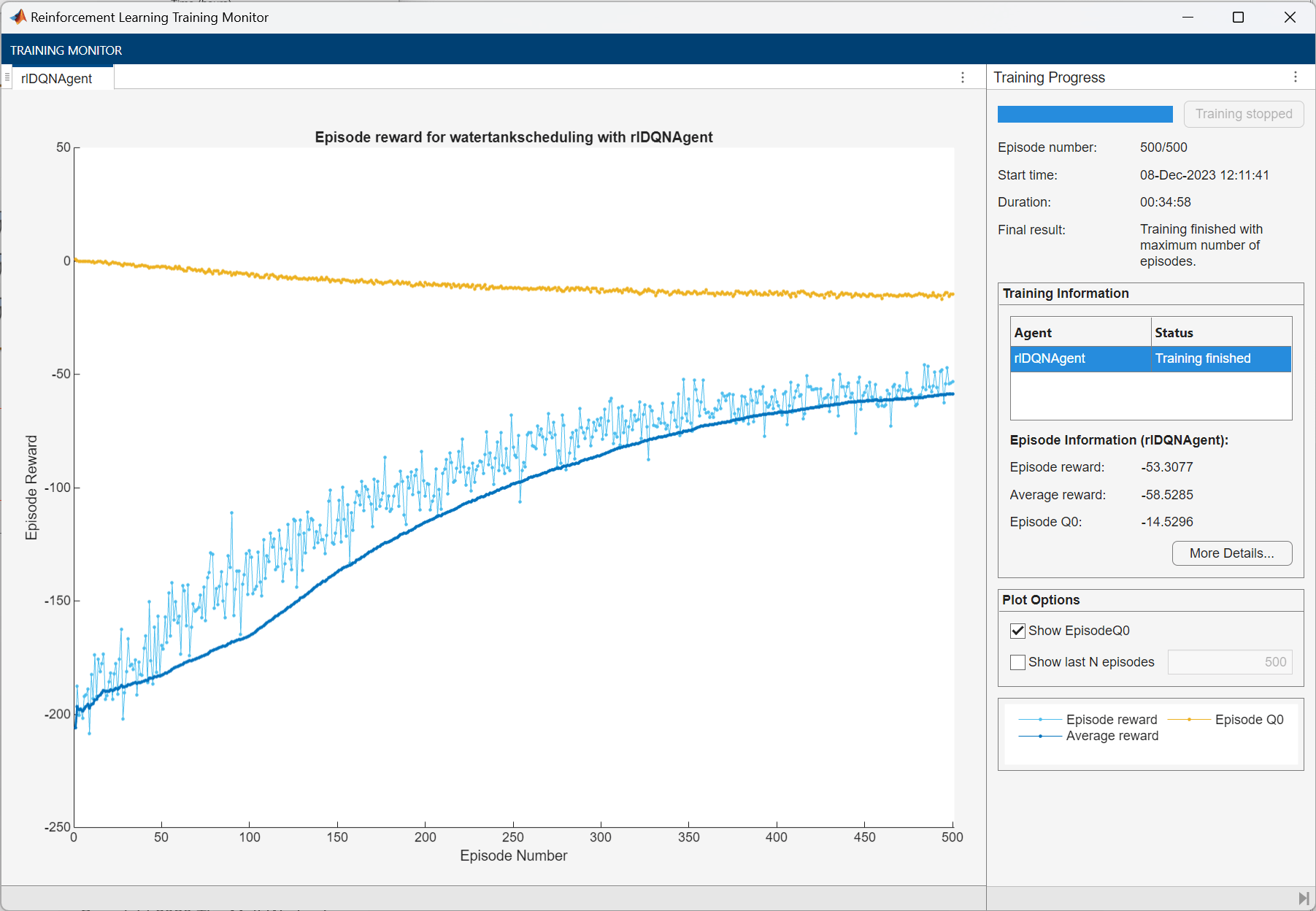### Simulate DQN Agent

To validate the performance of the trained agent, simulate it in the water-tank environment. For more information on agent simulation, see `rlSimulationOptions` and `sim`.

To simulate the agent performance, connect the RL Agent block by toggling the manual switch block.

`set_param(mdl+"/Manual Switch",'sw','0');`

Set the maximum number of steps for each simulaiton and the number of simulations. For this example, run 30 simulations. The environment reset function sets a different initial water height and water demand are different in each simulation.

```NumSimulations = 30; simOptions = rlSimulationOptions('MaxSteps',T_max/SampleTime,... 'NumSimulations', NumSimulations);```

To compare the agent with the baseline controller under the same conditions, reset the inital random seed used in the environment reset function.

`env.ResetFcn("Reset seed");`

Simulate the agent against the evironment.

`experienceDQN = sim(env,agent,simOptions);`

### Simulate Baseline Controller

To compare DQN agent with the baseline controller, you must simulate the baseline controller using the same simulation options and initial random seed for the reset function.

Enable the baseline controller.

`set_param(mdl+"/Manual Switch",'sw','1');`

To compare the agent with the baseline controller under the same conditions, reset the random seed used in the environment reset function.

`env.ResetFcn("Reset seed");`

Simulate the baseline controller against the environment.

`experienceBaseline = sim(env,agent,simOptions);`

### Compare DQN Agent with Baseline Controller

Initialize cumulative reward result vectors for both the agent and baseline controller.

```resultVectorDQN = zeros(NumSimulations, 1); resultVectorBaseline = zeros(NumSimulations,1);```

Calculate cumulative rewards for both the agent agent and baseline controller.

```for ct = 1:NumSimulations resultVectorDQN(ct) = sum(experienceDQN(ct).Reward); resultVectorBaseline(ct) = sum(experienceBaseline(ct).Reward); end```

Plot the cumulative rewards.

```plot([resultVectorDQN resultVectorBaseline],'o') set(gca,'xtick',1:NumSimulations) xlabel("Simulation number") ylabel('Cumulative Reward') legend('DQN','Baseline','Location','NorthEastOutside')```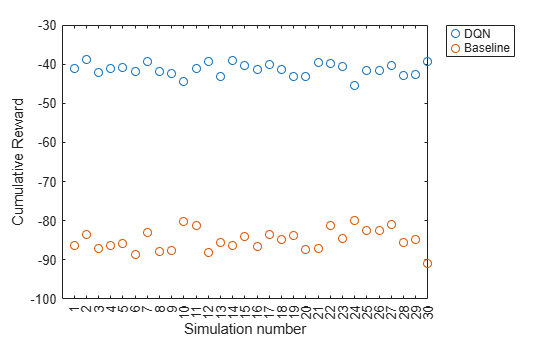The cumulative reward obtained by the agent is consistently around –40. This value is much greater than the average reward obtained by the baseline controller. Therefore, the DQN agent consistently outperforms the baseline controller in terms of energy savings.

### Local Functions

Water Demand Function

```function [WaterDemand,T_max] = generateWaterDemand(num_days) t = 0:(num_days*24)-1; % hr T_max = t(end); Demand_mean = [28, 28, 28, 45, 55, 110, 280, 450, 310, 170, 160, 145, 130, ... 150, 165, 155, 170, 265, 360, 240, 120, 83, 45, 28]'; % m^3/hr Demand = repmat(Demand_mean,1,num_days); Demand = Demand(:); % Add noise to demand a = -25; % m^3/hr b = 25; % m^3/hr Demand_noise = a + (b-a).*rand(numel(Demand),1); WaterDemand = timeseries(Demand + Demand_noise,t); WaterDemand.Name = "Water Demand"; end```

Reset Function

```function in = localResetFcn(in) % Use a persistent random seed value to evaluate the agent and the baseline % controller under the same conditions. persistent randomSeed if isempty(randomSeed) randomSeed = 0; end if strcmp(in,"Reset seed") randomSeed = 0; return end randomSeed = randomSeed + 1; rng(randomSeed) % Randomize water demand. num_days = 4; H_max = 7; [WaterDemand,~] = generateWaterDemand(num_days); assignin('base','WaterDemand',WaterDemand) % Randomize initial height. h0 = 3*randn; while h0 <= 0 || h0 >= H_max h0 = 3*randn; end blk = 'watertankscheduling/Water Tank System/Initial Water Height'; in = setBlockParameter(in,blk,'Value',num2str(h0)); end```